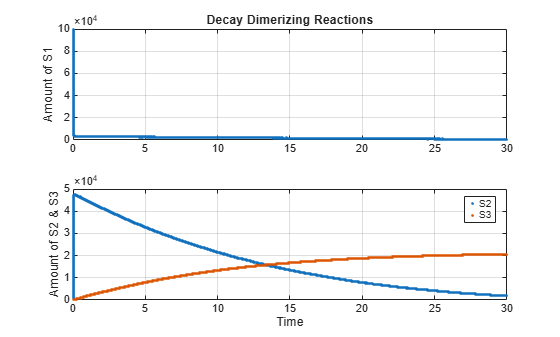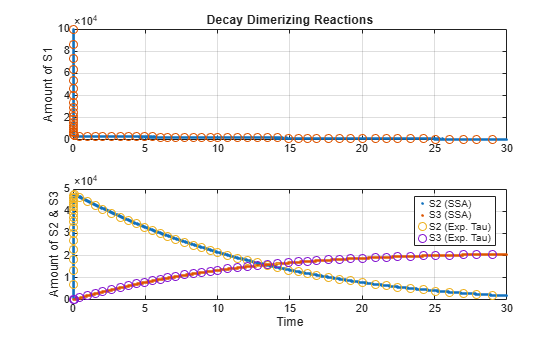# Comparing SSA and Explicit Tau-Leaping Stochastic Solvers

This example shows how to build and simulate a model using the SSA stochastic solver and the Explicit Tau-Leaping solver.

The following decaying-dimerizing reactions will be constructed and stochastically simulated:

• Reaction 1: s1 -> null, with reaction rate constant, c1 = 1.0

• Reaction 2: 2 s1 < - > s2, with reaction rate constants, forward: c2f = 0.002 reverse: c2r = 0.5

• Reaction 3: s2 -> s3, with reaction rate constant, c3 = 0.04

• Initial conditions: s1 = 100000 molecules, s2 = s3 = 0

This example uses parameters and conditions as described in Daniel T. Gillespie, 2001, "Approximate accelerated stochastic simulation of chemically reacting systems," Journal of Chemical Physics, vol. 115, no. 4, pp. 1716-1733.

### Create Decaying-Dimerizing Model

`model = sbiomodel('Decaying-Dimerizing Reaction Set');`

### Enter Reactions

```r1 = addreaction(model, 's1 -> null'); r2 = addreaction(model, '2 s1 <-> s2'); r3 = addreaction(model, 's2 -> s3');```

### Set Reactions to be MassAction

```kl1 = addkineticlaw(r1, 'MassAction'); kl2 = addkineticlaw(r2, 'MassAction'); kl3 = addkineticlaw(r3, 'MassAction');```

### Add Rate Constants for Each Reaction

```p1 = addparameter(kl1, 'c1', 'Value', 1.0); p2f = addparameter(kl2, 'c2f', 'Value', 0.002); p2r = addparameter(kl2, 'c2r', 'Value', 0.5); p3 = addparameter(kl3, 'c3', 'Value', 0.04);```

### Set the Kinetic Law Constants for Each Kinetic Law.

```kl1.ParameterVariableNames = {'c1'}; kl2.ParameterVariableNames = {'c2f', 'c2r'}; kl3.ParameterVariableNames = {'c3'};```

### Specify Initial Amounts of Each Species

```model.species(1).InitialAmount = 100000; % s1 model.species(2).InitialAmount = 0; % s2 model.species(3).InitialAmount = 0; % s3```

### Display the Completed Model Objects

`model`
```model = SimBiology Model - Decaying-Dimerizing Reaction Set Model Components: Compartments: 1 Events: 0 Parameters: 4 Reactions: 3 Rules: 0 Species: 3 Observables: 0 ```

### Display the Reaction Objects

`model.Reactions`
```ans = SimBiology Reaction Array Index: Reaction: 1 s1 -> null 2 2 s1 <-> s2 3 s2 -> s3 ```

### Display the Species Objects

`model.Species`
```ans = SimBiology Species Array Index: Compartment: Name: Value: Units: 1 unnamed s1 100000 2 unnamed s2 0 3 unnamed s3 0 ```

### Get the Active Configuration Set for the Model.

`cs = getconfigset(model,'active');`

### Simulate Model Using SSA Stochastic Solver and Plot

tfinal = 30, logging every 10th datapoint.

```cs.SolverType = 'ssa'; cs.StopTime = 30; solver = cs.SolverOptions; solver.LogDecimation = 10; cs.CompileOptions.DimensionalAnalysis = false; [t_ssa, x_ssa] = sbiosimulate(model); h1 = subplot(2,1,1); plot(h1, t_ssa, x_ssa(:,1),'.'); h2 = subplot(2,1,2); plot(h2, t_ssa, x_ssa(:,2:3),'.'); grid(h1,'on'); grid(h2,'on'); title(h1,'Decay Dimerizing Reactions'); ylabel(h1,'Amount of S1'); ylabel(h2,'Amount of S2 & S3'); xlabel(h2,'Time'); legend(h2, 'S2', 'S3');```### Simulate Model Using Explicit Tau-Leaping Solver and Plot in the Same Figure

Without closing the figure window, plot the results from using the Explicit Tau-Leaping Solver.

tfinal = 30, logging every 10th datapoint. Acceptable error tolerance for solver, 0.03.

```cs.StopTime = 30; cs.SolverType = 'explTau'; solver = cs.SolverOptions; solver.LogDecimation = 10; [t_etl, x_etl] = sbiosimulate(model); hold(h1,'on'); hold(h2,'on'); plot(h1, t_etl, x_etl(:,1),'o'); plot(h2, t_etl, x_etl(:,2:3),'o'); legend(h2, 'S2 (SSA)', 'S3 (SSA)', 'S2 (Exp. Tau)', 'S3 (Exp. Tau)'); hold(h1,'off'); hold(h2,'off');```### Comparison of Number of Steps for SSA and Explicit Tau-Leaping Algorithms

`fprintf('Approximate Number of SSA steps: %d\n', (length(t_ssa) * 10));`
```Approximate Number of SSA steps: 616010 ```
```fprintf('Approximate Number of Explicit Tau-Leaping steps: %d\n', ... (length(t_etl) * 10));```
```Approximate Number of Explicit Tau-Leaping steps: 620 ```